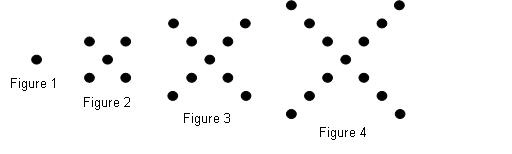Name:    Quiz 9.4-9.5

Multiple Choice
Identify the choice that best completes the statement or answers the question.

Which function does the graph represent?

1.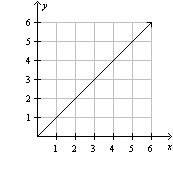a. y = 2x – 1 c. y = x + 1 b. y = 0.5x d. y = x

Numeric Response

1.

The graph of a function is a straight line that goes through the points (4, 1), (7, 7), and (9, y). What is the value of y?

Graph the data.

1.

 Input, x Output, y 2 2.5 4 3 6 3.5 8 4

Graph the function.

2.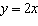Does the graph represent a linear function?  Explain.

3.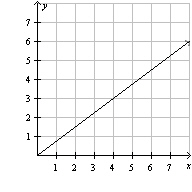4.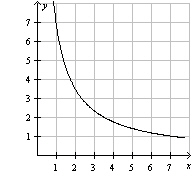5.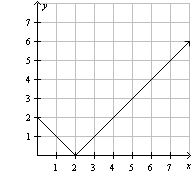6.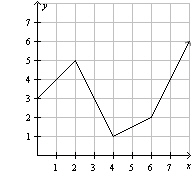Complete the table. Then make a graph of the data. Write an equation for the function.

7.

Find the cost of p pounds of peppers at a price of \$5 per pound.

 Pounds, p 1 2 3 4 Cost, c

Does the input-output table represent a linear function? Explain

8.

 Input, x 1 2 3 4 Output, y 5 9 13 17

9.

 Input, x 1 5 9 13 Output, y 13 17 21 17

Make an input-output table for the pattern. Is the function relating the figure number x to the number of dots y linear? Explain.

10.